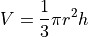# 4.7. Functions, loops, and types assignment¶

Note

This assignment is available as an Ipython notebook  (.ipynb)

In this assignment we review basic concepts of containers, loops, and functions.

## 4.7.1. Exercise 1¶

We do the first exercise as an example. Suppose:

>>> a_list = [2,7,8, 19]
2


The idea is to write the line of code that will produce the given output, 2 in this case. It should this be an expression that operates on a_list. A simple correct answer is:

>>> a_list = [2,7,8, 19]
>>> a_list
2


## 4.7.2. Exercise 2¶

Suppose:

>>> a_list = [2,7,8, 19]
19


Write the line of code that produces the given output.

## 4.7.3. Exercise 3¶

Suppose:

>>> a_list = [2,7,8, 19]
[7,8]


Write the line of code that produces the given output.

## 4.7.4. Exercise 4¶

Suppose:

>>> a_list = [2,7,8, 19]
[3,8,9,20]


Write the line of code that produces the given output. [Hint: Try a list comprehension]

## 4.7.5. Exercise 5¶

Suppose:

>>> a_list = [2,7,8, 19]
[True, True, False, False]


Write the line of code that produces the given output. [Hint: Try a list comprehension that collects a list of the results of a Boolean test]

## 4.7.6. Exercise 6¶

Write a for loop that collects all the words in a list that are three letters long and places them in a list named result.

Here is a list to work on:

>>> test_list = ['the', 'be', 'of', 'and', 'a', 'in', 'to', 'have', 'it',
'to', 'for', 'I', 'that', 'you', 'he', 'on', 'with', 'do',
'at', 'by', 'not', 'this', 'but', 'from', 'they', 'his']


Note This can be done as a list comprehension and you are welcome to do it that way.

## 4.7.7. Exercise 7¶

Now, using the same test_list as in exercise 6, write a for loop that counts the number of words that are three letters long in test_list and places the result in a variable named three_let_word_freq. You can do this by just taking the length of the list you computed in exercise 7, but try to do it without building that list. Just use a variable whose value is an integer representing the number of three letter words seen thus far and increment it by one each time you see a three-letter word.

## 4.7.8. Exercise 8¶

Write a for loop that finds the item which occurs most frequently in a list of items. You can use the following list as an example:

L = ['1', '4', '1', '5', '9', '2', '6', '5', '3', '5',
'8', '9', '7', '9', '3', '1', '1', '6']


You will need a dictionary and it will be most convenient to use a Counter. It will be helpful to review our discussion of Counters in Section Dictionaries. It will also be helpful to read the Python docs to find a convenient method that finds the element in a counter with the highest count.

## 4.7.9. Exercise 9¶

Write a function that adds 1 to any number. Here is how it should work:

>>> x = add1(2)
>>> x
3


Hint: If you’re having trouble getting this behavior, maybe you forgot about return?

## 4.7.10. Exercise 10¶

Write a function that converts temperatures (as numbers) from Fahrenheit to Celsius scale. Here is how it should work:

>>>  C = fahrenheit_to_celsius(212)
>>>  C
100.0
>>>  C = fahrenheit_to_celsius(-40)
>>>  C
-40.0
>>>  C = fahrenheit_to_celsius(32)
>>>  C
0.0


Write a function that converts temperatures (as numbers) from Fahrenheit to Celsius scale. Here is how it should work:

>>>  C = fahrenheit_to_celsius(212)
>>>  C
100.0
>>>  C = fahrenheit_to_celsius(-40)
>>>  C
-40.0
>>>  C = fahrenheit_to_celsius(32)
>>>  C
0.0


## 4.7.11. Exercise 11¶

Write a function that computes the volume of a cone. Note that if V is the volume of a cone, and h is its height and r is the radius of the base, thenHere is how the function should work:

>>>  V = cone_volume(2,3)
>>>  V
37.69911184307752